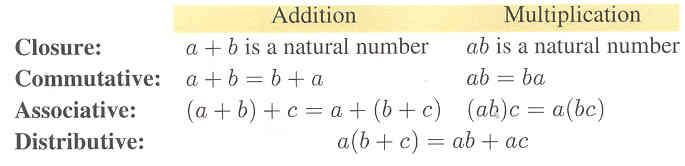# Section 5.1: Natural Numbers

## 5.1 Outline

1. Basic concepts
1. counting numbers
2. natural numbers
2. Closure property
1. definition
2. closed/not closed
3. Commutative and associative properties
3. definition of multiplication
4. closure for multiplication in the set of natural numbers
5. commutative property for multiplication
6. associative property for multiplication
4. Distributive property
5. Definition of subtraction

## 5.1 Essential Ideas

The basics of arithmetic and algebra and presented in this section. We assume knowledge of the natural numbers (also known as the counting numbers) and the basic operation of addition. Given this assumption, we define multiplication (as repeated addition), subtraction (as an addition), and division (as a multiplication). There are are some self-evident properties. Let a and b be natural numbers; then,There are other fundamental properties identity and inverse which will be defined later in this chapter.
Definition of Subtraction

The operation of subtraction is defined in terms of addition: a − b = x means a = b + x